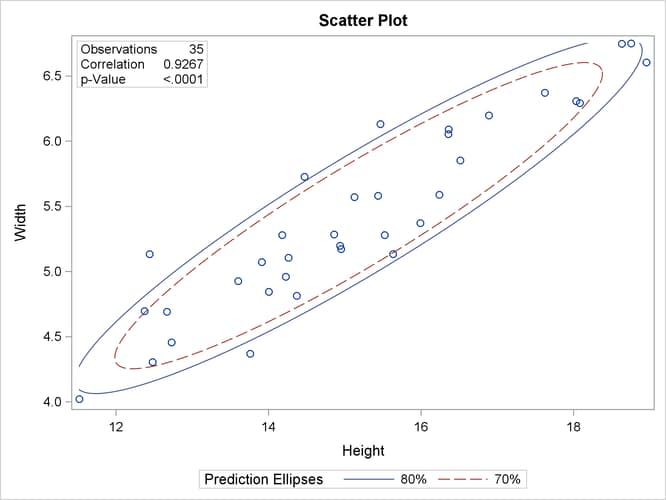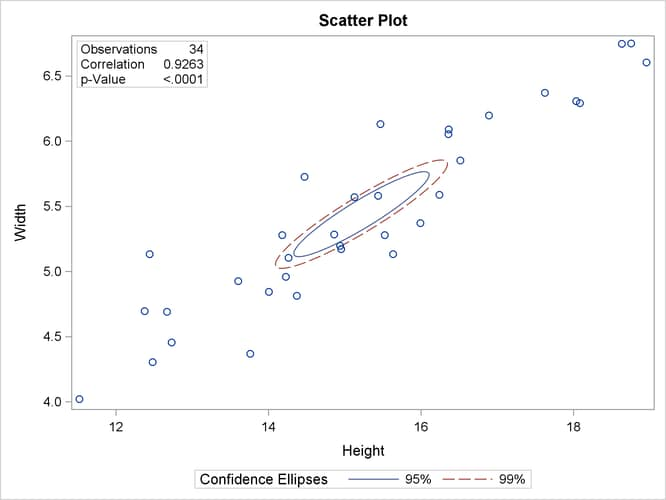# The CORR Procedure

### Example 2.8 Creating Scatter Plots

The following statements request a correlation analysis and a scatter plot matrix for the variables in the data set `Fish1`, which was created in Example 2.6.

```ods graphics on;
title 'Fish Measurement Data';
proc corr data=fish1 nomiss plots=matrix(histogram);
var Height Width Length3 Weight3;
run;
```

The "Simple Statistics" table in Output 2.8.1 displays univariate descriptive statistics for analysis variables.

Output 2.8.1: Simple Statistics

 Fish Measurement Data

The CORR Procedure

4 Variables: Height Width Length3 Weight3

Simple Statistics
Variable N Mean Std Dev Sum Minimum Maximum
Height 34 15.22057 1.98159 517.49950 11.52000 18.95700
Width 34 5.43805 0.72967 184.89370 4.02000 6.74970
Length3 34 38.38529 4.21628 1305 30.00000 46.50000
Weight3 34 8.44751 0.97574 287.21524 6.23168 10.00000

The "Pearson Correlation Coefficients" table in Output 2.8.2 displays Pearson correlation statistics for pairs of analysis variables.

Output 2.8.2: Pearson Correlation Coefficients

Pearson Correlation Coefficients, N = 34
Prob > |r| under H0: Rho=0
Height Width Length3 Weight3
Height
 1.00000
 0.92632 <.0001
 0.95492 <.0001
 0.96261 <.0001
Width
 0.92632 <.0001
 1.00000
 0.92171 <.0001
 0.92789 <.0001
Length3
 0.95492 <.0001
 0.92171 <.0001
 1.00000
 0.96523 <.0001
Weight3
 0.96261 <.0001
 0.92789 <.0001
 0.96523 <.0001
 1.00000

The variables are highly correlated. For example, the correlation between `Height` and `Width` is 0.92632.

The PLOTS=MATRIX(HISTOGRAM) option requests a scatter plot matrix for the VAR statement variables in Output 2.8.3.

Output 2.8.3: Scatter Plot MatrixNote that this graphical display is requested by enabling ODS Graphics and by specifying the PLOTS= option. For more information about ODS Graphics, see Chapter 21: Statistical Graphics Using ODS in SAS/STAT 14.2 User's Guide.

To explore the correlation between `Height` and `Width`, the following statements display (in Output 2.8.4) a scatter plot with prediction ellipses for the two variables:

```ods graphics on;
proc corr data=fish1 nomiss
plots=scatter(nvar=2 alpha=.20 .30);
var Height Width Length3 Weight3;
run;
```

The PLOTS=SCATTER(NVAR=2) option requests a scatter plot for the first two variables in the VAR list. The ALPHA=.20 .30 suboption requests and prediction ellipses, respectively.

Output 2.8.4: Scatter Plot with Prediction EllipsesA prediction ellipse is a region for predicting a new observation from the population, assuming bivariate normality. It also approximates a region that contains a specified percentage of the population. The displayed prediction ellipse is centered at the means . For further details, see the section Confidence and Prediction Ellipses.

Note that the following statements also display (in Output 2.8.5) a scatter plot for `Height` and `Width`:

```ods graphics on;
proc corr data=fish1
plots=scatter(alpha=.20 .30);
var Height Width;
run;
```

Output 2.8.5: Scatter Plot with Prediction EllipsesOutput 2.8.5 includes the point , which was excluded from Output 2.8.4 because the observation had a missing value for `Weight3`. The prediction ellipses in Output 2.8.5 also reflect the inclusion of this observation.

The following statements display (in Output 2.8.6) a scatter plot with confidence ellipses for the mean:

```ods graphics on;
title 'Fish Measurement Data';
proc corr data=fish1 nomiss
plots=scatter(ellipse=confidence nvar=2 alpha=.05 .01);
var Height Width Length3 Weight3;
run;
```

The NVAR=2 suboption within the PLOTS= option restricts the number of plots created to the first two variables in the VAR statement, and the ELLIPSE=CONFIDENCE suboption requests confidence ellipses for the mean. The ALPHA=.05 .01 suboption requests and confidence ellipses, respectively.

Output 2.8.6: Scatter Plot with Confidence EllipsesThe confidence ellipse for the mean is centered at the means . For further details, see the section Confidence and Prediction Ellipses.